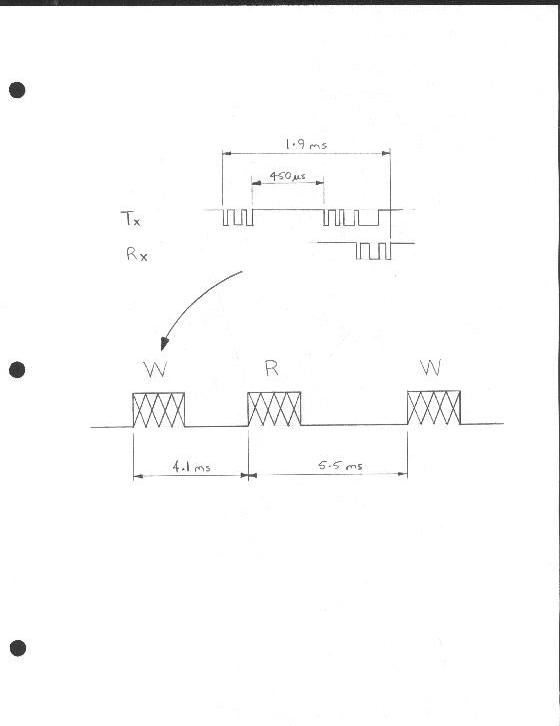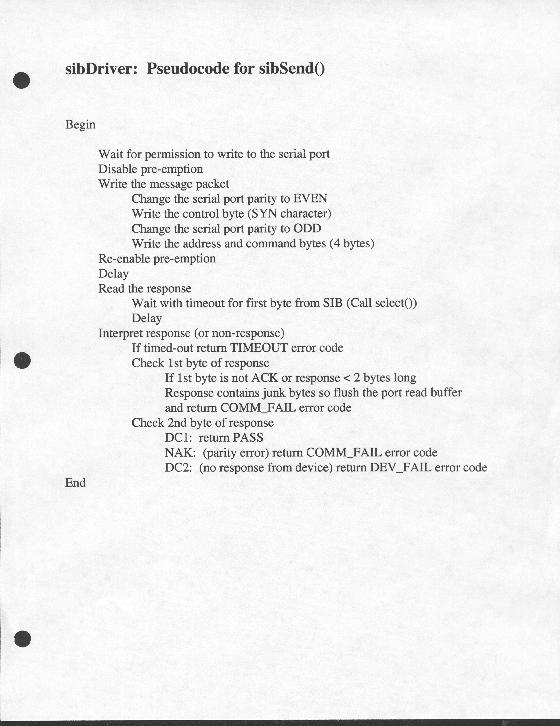NRAO Real-time Programmers Meeting 12 April 1999

The New Standard Interface Board Library
for
GBT Monitor & Control
Simon Hoyle
John Ford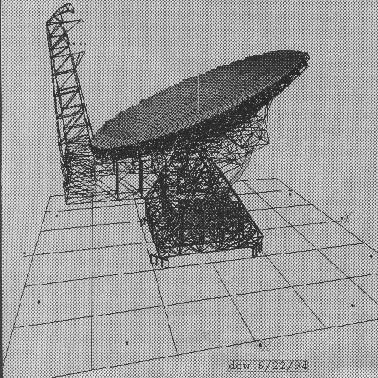NRAO Real-time Programmers Meeting 12 April 1999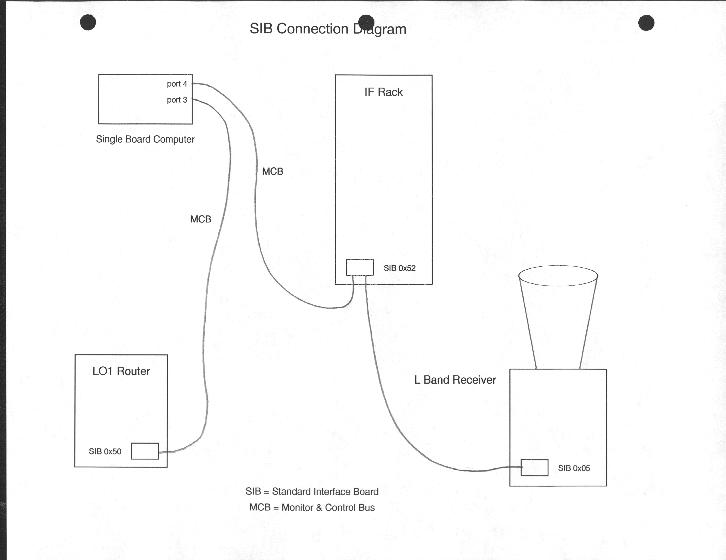#### WHAT

* The GBT uses many VLBA Standard Interface Boards for device control and monitor, where device may be
Converter Rack, L Band Receiver, Switching Signal Selector etc.

* A Standard Interface Board (SIB) allows a single board computer (SBC) to issue digital commands to a device
and read digital and analog data.

* An SBC communicates with a number of SIBs via an RS485 serial cable.

* One or more serial cables connected to one or more of the SBC's serial ports are treated as one logical bus,
the Monitor and Control Bus (MCB).

* The Sib library provides methods for GBT software device Managers to open, read and write to an SIB.  The library
takes care of SIB initialization, protocol, timing and data synchronization issues.

* The NEW Sib Library replaces a version that has been in use for the past 5 or so years.

NRAO Real-time Programmers Meeting 12 April 1999

#### WHY

* To stop client tasks from wasting cpu time and MCB bandwidth in trying to access a dead SIB/Device.

* To provide improved error detection and reporting to client programs.

* To recover from power outage and restore control values without the intervention of upper software levels.

* To make it easier to accomodate hardware or software changes by using a simple, hierarchial software
architecture.

#### AIMS

+ Make efficient use of the bus

+ Use the OS provided tty driver if possible

+ Allow low priority tasks to access the MCB without unduly affecting the established
priority order.

+ Retain the upper level interface.

+ Implement the lower level as a VxWorks device driver.

+ Return simple, meaningful error codes to the client program.

+ Preserve the multi, non-preassigned port feature.

+ Maintain synchrony between hardware and software.

+ Detect and recover from errors eg power outage, power cycle, bad communications path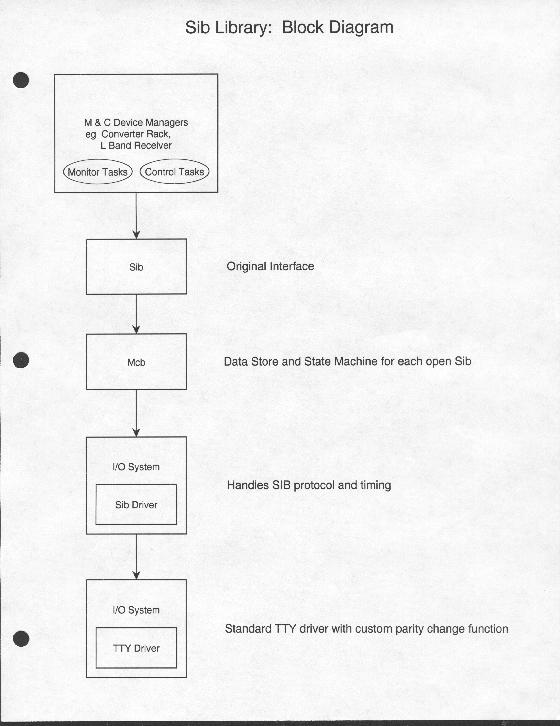NRAO Real-time Programmers Meeting 12 April 1999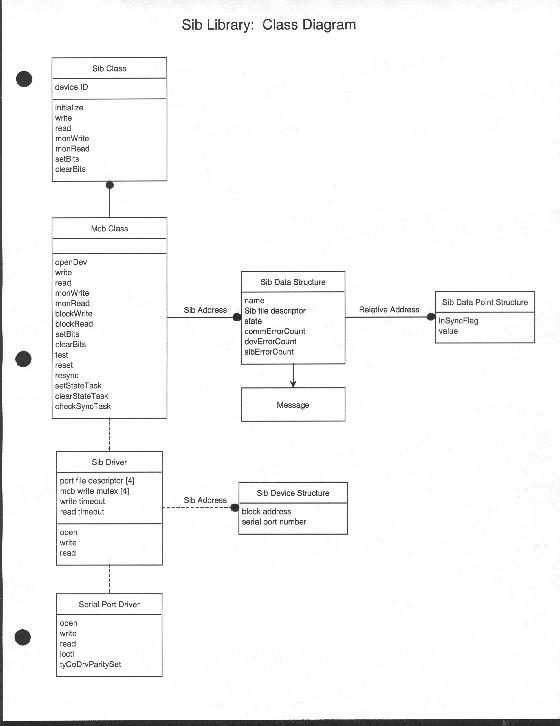#### HOW

* Simple, 3 layer hierarchy

+ Sib Class preserves original interface
+ Mcb Class maintains state information and data store for each open SIB
+ Sib Driver handles SIB communication protocol and timing.  It configures
the serial port(s) and keeps track of port allocation for each open SIB.

* Sib Class

+ Mostly just interface to Mcb Class
+ M & C device managers instantiate one per device typically.

* Mcb Class

+ Data store for each open SIB
- Error counters
- State information:
- List of all SIB relative addresses that have been successfully read/written and
the data value.
+ Read and write methods return an error code without attempting to talk to the hardware
if an SIB's state is not READY
Regularly examines the error counters of each Sib and changes the state
if any of the error counter values exceed preset levels
Regularly examines the state of each Sib and changes the state back to READY
if the SIB responds to a read.  In the case of SIB_OFF->READY it re-initializes
the SIB and re-writes all the control values.
Low priority task that regularly reads control RAs and compares with the values
stored in software.  It flags and tries to re-write any that are found to be Not In Sync.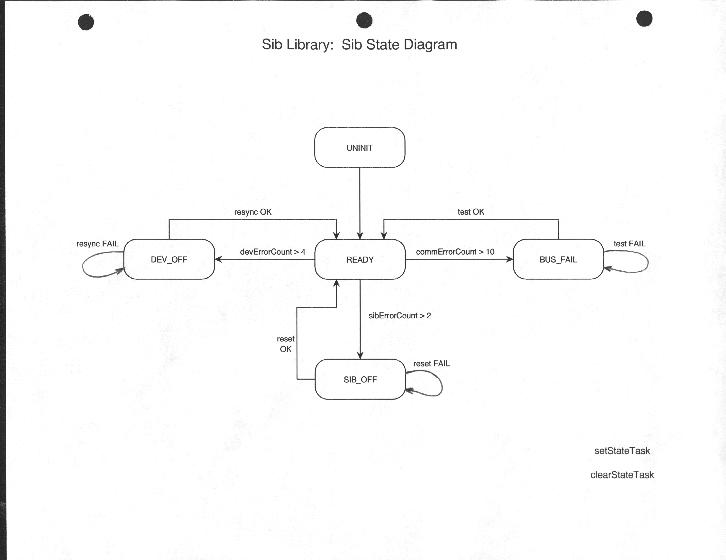* Sib Driver

+ Written as a VxWorks I/O system driver
- open
Configures serial port(s), searches for, and initializes an SIB.
+ Calls standard tty driver with timeout
- Uses select() for port read timeout
Select provides wait with timeout on multiple file descriptors.
+ Handles protocol and timing
+ Interprets response from SIB and returns an error code as appropriate.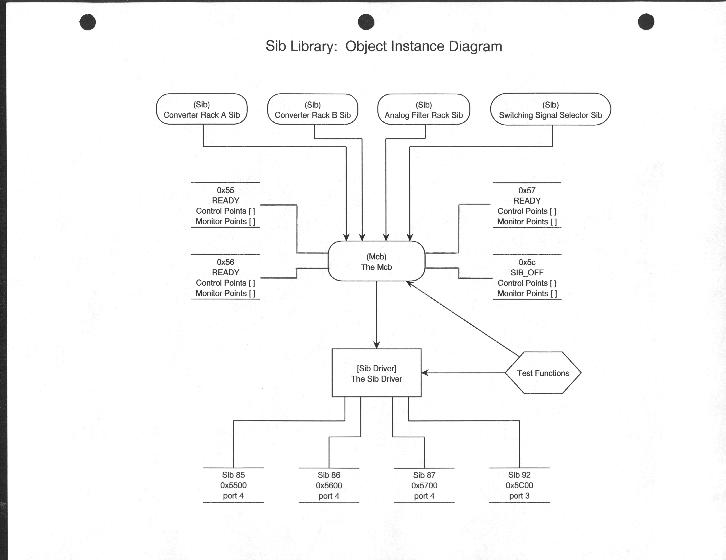NRAO Real-time Programmers Meeting 12 April 1999
###### Target Shell Commands
-> mcbSearch

Searching .........................................
.........................................
Found Sib device at address 0x50
.
Found Sib device at address 0x52
.....
Found Sib device at address 0x58
...........
Found Sib device at address 0x64
...........................

-> DUT = 0x64

Sib device 0x64: Value at RA 50 = 0xffc0

Sib device 0x64:
1) Value at RA a = 0x727f
2) Value at RA a = 0x727f
3) Value at RA a = 0x727f
4) Value at RA a = 0x727f
5) Value at RA a = 0x727f

-> mcbPrintErrors

Sib device 0x64:
Control data parity error counter, messages in block = 0
Invalid SYN character count = 0
Control data parity error counter, all messages = 0
Address parity error counter, all messages = 0
No monitor response counter = 0
No control response counter = 0

-> printMcbInfo

There are 5 open SIB devices.

Name: sib5           Address: 0x5              State: SIB_OFF
commErrorCount = 0              devErrorCount = 0              sibErrorCount = 12
controlPoints:
RA 40 = 0x866 NIS   RA 41 = 0x800 NIS   RA 48 = 0x7 NIS     RA 49 = 0xffcc NIS
RA 4b = 0x0 NIS       RA 4c = 0x6 NIS      RA 4d = 0xfff7 NIS   RA 4e = 0xfff7 NIS
monitorPoints:
RA 0 = 0x5eaf      RA 4 = 0xeadf       RA 5 = 0xcc0f        RA 6 = 0xd02f
RA 7 = 0x70ff       RA 8 = 0x7eff        RA 9 = 0x607f        RA a = 0xa65f
RA b = 0x3e6f      RA c = 0x58ef       RA d = 0x586f        RA e = 0x5a5f
RA 16 = 0x5c3f     RA 17 = 0x58ef     RA 25 = 0x800f      RA 26 = 0x82af
RA 27 = 0x800f     RA 28 = 0xc1f       RA 29 = 0x800f      RA 2a = 0xfc1f
RA 2e = 0x49f       RA 40 = 0xf866     RA 41 = 0xf800      RA 48 = 0xfcf7
RA 49 = 0xfccc     RA 4b = 0xfc00     RA 4c = 0xfcf6       RA 4d = 0xfcf7
RA 4e = 0xfcf7      RA 50 = 0xfc5f      RA 52 = 0xfcff        RA 53 = 0xfcfc

commErrorCount = 0              devErrorCount = 0              sibErrorCount = 0
controlPoints:
RA 0 = 0xfffe       RA 1 = 0xfffe       RA 2 = 0xfffd       RA 3 = 0xfffe
RA 4 = 0xffef       RA 5 = 0xffff        RA 6 = 0xffff        RA 7 = 0xffff
RA 8 = 0xfffe       RA 9 = 0xfffe       RA a = 0x1         RA 10 = 0xfffe
RA 11 = 0xfff7     RA 12 = 0xfff7      RA 13 = 0xffff      RA 14 = 0xffff
monitorPoints:
RA 0 = 0xfffe        RA 1 = 0xfffe       RA 2 = 0xfffd       RA 3 = 0xfffe
RA 4 = 0xffef        RA 5 = 0xffff        RA 6 = 0xffff        RA 7 = 0xffff
RA 8 = 0xfffe        RA 9 = 0xfffe       RA a = 0xff01      RA 10 = 0xfffe
RA 11 = 0xfff7      RA 12 = 0xfff7      RA 13 = 0xffff      RA 14 = 0xffff
RA 18 = 0xfffe      RA 19 = 0xfffe      RA 28 = 0xfffe

commErrorCount = 0              devErrorCount = 0              sibErrorCount = 0
controlPoints:
RA 20 = 0x7         RA 21 = 0x7          RA 22 = 0x7         RA 23 = 0x7
RA 24 = 0x7         RA 25 = 0x7          RA 26 = 0x7         RA 27 = 0x7
RA 28 = 0x0         RA 29 = 0x49        RA 2a = 0x49        RA 2b = 0x0
RA 2c = 0x0         RA 2d = 0x5b        RA 2e = 0x5b        RA 2f = 0x0
RA 30 = 0x0
monitorPoints:
RA 0 = 0xff5f         RA 1 = 0x6ef       RA 2 = 0x48f         RA 3 = 0xef
RA 8 = 0xfdff         RA 9 = 0xffff        RA a = 0xffff          RA b = 0xffff
RA 10 = 0xf42f      RA 11 = 0xf2ff      RA 12 = 0xf8af      RA 13 = 0xf9af
RA 14 = 0xfeff       RA 15 = 0xfe3f     RA 16 = 0xffdf       RA 17 = 0x21f
RA 1b = 0x416f     RA 1c = 0xbebf    RA 1d = 0x60af     RA 1e = 0x9f1f
RA 20 = 0xff07      RA 21 = 0xff07     RA 22 = 0xff07      RA 23 = 0xff07
RA 24 = 0xff07      RA 25 = 0xff07     RA 26 = 0xff07      RA 27 = 0xff07
RA 28 = 0xff00      RA 29 = 0xff49     RA 2a = 0xff49      RA 2b = 0xff00
RA 2c = 0xff00      RA 2d = 0xff5b     RA 2e = 0xff5b      RA 2f = 0xff00
RA 30 = 0xff00      RA 31 = 0xffff       RA 32 = 0xffff

commErrorCount = 0              devErrorCount = 0              sibErrorCount = 0
controlPoints:
RA 48 = 0x3 NIS
monitorPoints:
RA 48 = 0xfff3

commErrorCount = 0              devErrorCount = 0              sibErrorCount = 0
controlPoints:
RA 49 = 0x1
monitorPoints:
RA 0 = 0x1fef        RA 1 = 0x36df       RA 2 = 0x727f        RA 3 = 0xffef
RA 4 = 0x727f       RA 5 = 0xffff          RA 6 = 0x727f        RA 7 = 0xffef
RA 8 = 0x727f       RA 9 = 0xffef         RA a = 0x727f        RA b = 0xffff
RA c = 0xd3bf       RA d = 0xdf2f        RA e = 0xd6bf        RA f = 0xdeaf
RA 10 = 0x685f     RA 11 = 0x69df      RA 12 = 0x69ef      RA 13 = 0x69df
RA 49 = 0xff01      RA 50 = 0xffc0       RA 51 = 0xdf64      RA 52 = 0xffdf
RA 53 = 0xffdf       RA 54 = 0xffdf        RA 55 = 0xfff7

NRAO Real-time Programmers Meeting 12 April 1999

#### Real time

* Priority and pre-emption
Priority of the calling task is preserved and pre-emption is allowed until deep in the Sib Driver.

* Resource contention
Mutex semaphores to control serial port access - one per port.
FIFO queuing chosen so that lower priority tasks get fair access.

* Implementing small delays

#### Hardware

* Parity change and timing
Need to wait until tty driver has finished writing before changing the parity - otherwise
data bytes become corrupted.

* Spurious bytes on bus
Powering down or disconnecting the cable from an SIB generates a spurious byte on the bus which the
tty driver dutifully stores in the buffer.
Need to detect and discard message packet containing junk byte(s) or preferably just discard the offending
byte(s).

#### Performance

* Small loss in maximum communication rate
35% to 46% efficiency in terms of bandwidth usage.

* Gains at application task level
Minimal time lost on dead SIBs/Devices.
Concurrent port access.
Single write operation to change particular bits.

NRAO Real-time Programmers Meeting 12 April 1999

#### TIMING DIAGRAM

| odd parity |      |          even parity          |